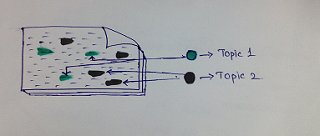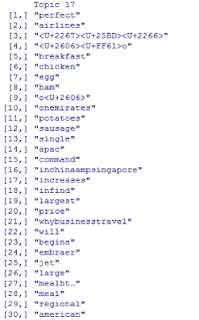# BigDataNews

A Data Science Central Community

As a part of Twitter Data Analysis, So far I have completed Movie review using RDocument Classification using RToday we will be dealing with discovering topics in Tweets, i.e. to mine the tweets data to discover underlying topics– approach known as Topic Modeling.

What is Topic Modeling?
A statistical approach for discovering “abstracts/topics” from a collection of text documents based on statistics of each word. In simple terms, the process of looking into a large collection of documents, identifying clusters of words and grouping them together based on similarity and identifying patterns in the clusters appearing in multitude.
Consider the below Statements:
1. I love playing cricket.
2. Sachin is my favorite cricketer.
3. Titanic is heart touching movie.
4. Data Analytics is next Future in IT.
5. Data Analytics & Big Data complements each other.
When we apply Topic Modeling to the above statements, we will be able to group statement 1&2 as Topic-1 (later we can identify that the topic is Sport),statement 3 as Topic-2 (topic is Movies)statement 4&5 as Topic-3 (topic isdata Analytics).fig: Identifying topics in Documents and classifying as Topic 1 & Topic 2
Latent Dirichlet Allocation algorithm (LDA):
Topic Modeling can be achieved by using Latent Dirichlet Allocation algorithm. Not going into the nuts & bolts of the Algorithm, LDA automatically learns itself to assign probabilities to each & every word in the Corpus and classify into Topics. A simple explanation for LDA could be found here:
Steps Involved:
1. Fetch tweets data using ‘twitteR’ package.
2. Load the data into the R environment.
3. Clean the Data to remove: re-tweet information, links, special characters, emoticons, frequent words like is, as, this etc.
4. Create a Term Document Matrix (TDM) using ‘tm Package.
5. Calculate TF-IDF i.e. Term Frequency Inverse Document Frequency for all the words in word matrix created in Step 4.
6. Exclude all the words with tf-idf <= 0.1, to remove all the words which are less frequent.
7. Calculate the optimal Number of topics (K) in the Corpus using log-likelihood method for the TDM calculated in Step6.
8. Apply LDA method using topicmodels Package to discover topics.
9. Evaluate the model.Conclusion:
Topic modeling using LDA is a very good method of discovering topics underlying. The analysis will give  good results if and only if we have large set of Corpus.In the above analysis using tweets from top 5 Airlines, I could find that one of the topics which people are talking about is about FOOD being served. We can Sentiment Analysis techniques to mine what people thinks about, talks about products/companies etc.

SourceCode:
library("tm")

library("wordcloud")
library("slam")
library("topicmodels")
con <- file("tweets.txt", "rt")
#Clean Text
tweets = gsub("(RT|via)((?:\\b\\W*@\\w+)+)","",tweets)
tweets = gsub("http[^[:blank:]]+", "", tweets)
tweets = gsub("@\\w+", "", tweets)
tweets = gsub("[ \t]{2,}", "", tweets)
tweets = gsub("^\\s+|\\s+\$", "", tweets)
tweets <- gsub('\\d+', '', tweets)
tweets = gsub("[[:punct:]]", " ", tweets)
corpus = Corpus(VectorSource(tweets))
corpus = tm_map(corpus,removePunctuation)
corpus = tm_map(corpus,stripWhitespace)
corpus = tm_map(corpus,tolower)
corpus = tm_map(corpus,removeWords,stopwords("english"))
tdm = DocumentTermMatrix(corpus) # Creating a Term document Matrix
# create tf-idf matrix
term_tfidf <- tapply(tdm\$v/row_sums(tdm)[tdm\$i], tdm\$j, mean) * log2(nDocs(tdm)/col_sums(tdm > 0))
summary(term_tfidf)
tdm <- tdm[,term_tfidf >= 0.1]
tdm <- tdm[row_sums(tdm) > 0,]
summary(col_sums(tdm))
#Deciding best K value using Log-likelihood method
best.model <- lapply(seq(2, 50, by = 1), function(d){LDA(tdm, d)})
best.model.logLik <- as.data.frame(as.matrix(lapply(best.model, logLik)))
#calculating LDA
k = 50;#number of topics
SEED = 786; # number of tweets used
CSC_TM <-list(VEM = LDA(tdm, k = k, control = list(seed = SEED)),VEM_fixed = LDA(tdm, k = k,control = list(estimate.alpha = FALSE, seed = SEED)),Gibbs = LDA(tdm, k = k, method = "Gibbs",control = list(seed = SEED, burnin = 1000,thin = 100, iter = 1000)),CTM = CTM(tdm, k = k,control = list(seed = SEED,var = list(tol = 10^-4), em = list(tol = 10^-3))))
#To compare the fitted models we first investigate the values of the models fitted with VEM and estimated and with VEM and fixed
sapply(CSC_TM[1:2], slot, "alpha")
sapply(CSC_TM, function(x) mean(apply(posterior(x)\$topics, 1, function(z) - sum(z * log(z)))))
Topic <- topics(CSC_TM[["VEM"]], 1)
Terms <- terms(CSC_TM[["VEM"]], 8)
Terms

Originally posted here

Views: 15464

Tags: R, algorithm, learning, machine, modelling, topic

Comment